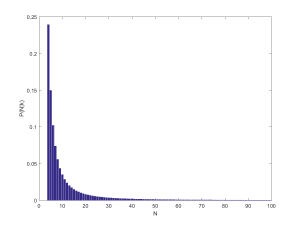How small is the wiki?

Recently I encountered a specialist Wiki. I pressed “random page” a few times, and got a repeat page after 5 tries. How many pages should I expect this small wiki to have?

We can compare this to the German tank problem. Note that it is different; in the tank problem we have a maximum sample (maybe like the web pages on the site were numbered), while here we have number of samples before repetition.

We can of course use Bayes theorem for this. If I get a repeat after$k$ random samples, the posterior distribution of$N$, the number of pages, is$P(N|k) = P(k|N)P(N)/P(k)$.

If I randomly sample from$N$ pages, the probability of getting a repeat on my second try is$1/N$, on my third try$2/N$, and so on:$P(k|N)=(k-1)/N$. Of course, there has to be more pages than$k-1$, otherwise a repeat must have happened before step$k$, so this is valid for$k \leq N+1$. Otherwise,$P(k|N)=0$ for$k>N+1$.

The prior$P(N)$ needs to be decided. One approach is to assume that websites have a power-law distributed number of pages. The majority are tiny, and then there are huge ones like Wikipedia; the exponent is close to 1. This gives us$P(N) = N^{-\alpha}/\zeta(\alpha)$. Note the appearance of the Riemann zeta function as a normalisation factor.

We can calculate$P(k)$ by summing over the different possible$N$:$P(k)=\sum_{N=1}^\infty P(k|N)P(N) = \frac{k-1}{\zeta(\alpha)}\sum_{N=k-1}^\infty N^{-(\alpha+1)}$$=\frac{k-1}{\zeta(\alpha)}(\zeta(\alpha+1)-\sum_{i=1}^{k-2}i^{-(\alpha+1)})$.

Putting it all together we get$P(N|k)=N^{-(\alpha+1)}/(\zeta(\alpha+1) -\sum_{i=1}^{k-2}i^{-(\alpha+1)})$ for$N\geq k-1$. The posterior distribution of number of pages is another power-law. Note that the dependency on$k$ is rather subtle: it is in the support of the distribution, and the upper limit of the partial sum.

What about the expected number of pages in the wiki?$E(N|k)=\sum_{N=1}^\infty N P(N|k) = \sum_{N=k-1}^\infty N^{-\alpha}/(\zeta(\alpha+1) -\sum_{i=1}^{k-2}i^{-(\alpha+1)})$$=\frac{\zeta(\alpha)-\sum_{i=1}^{k-2} i^{-\alpha}}{\zeta(\alpha+1)-\sum_{i=1}^{k-2}i^{-(\alpha+1)}}$. The expectation is the ratio of the zeta functions of$\alpha$ and$\alpha+1$, minus the first$k-2$ terms of their series.Distribution of P(N|5) for $\alpha=1.1$.

So, what does this tell us about the wiki I started with? Assuming$\alpha=1.1$ (close to the behavior of big websites), it predicts$E(N|k)\approx 21.28$. If one assumes a higher$\alpha=2$ the number of pages would be 7 (which was close to the size of the wiki when I looked at it last night – it has grown enough today for k to equal 13 when I tried it today).

So, can we derive a useful rule of thumb for the expected number of pages? Dividing by$k$ shows that$E(N|k)$ approaches proportionality, especially for larger$\alpha$:

So a good rule of thumb is that if you get$k$ pages before a repeat, expect between$2k$ and$4k$ pages on the site. However, remember that we are dealing with power-laws, so the variance can be surprisingly high.

2 thoughts on “How small is the wiki?”

1.Owen Cotton-Barratt says:

Taking the prior according to distribution of websites seems to assume that you drew your wiki randomly from the set of wikis. But larger wikis are probably linked to much more, so you’re disproportionately likely to find yourself on one.

I’m not sure exactly how to integrate this into the prior, but I expect the effect will be to reduce the effect of the prior, and move you closer to quadratic dependence on k.

2.Owen Cotton-Barratt says:

Ah, you say:

P(k|N) = (k – 1)/N

But I think this is the *conditional* probability of getting a repeat at step k *given* no earlier repeats. To get the probability of a first repeat at step k, you need to multiply by the probability of not getting any repeats until then. That is:

P(k|N) = (k-1)*(N)*(N-1)*(N-2)*…*(N-k+2)/N^k